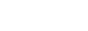## When does : (amend) not modify its first argument?

Short answer: When 1) projected or 2) modified by an adverb, e.g., :/: :\: or :’

Normally, the : (amend) function is used to assign a value to a name:

```q)foo: 47
q)foo
47
q)
```

Like other built-in functions that take two arguments, : (amend) can be called using either infix notation (as above) or function call notation:

```q):[foo; 747]
q)foo
747
q)
```

Note that : (amend) displays its special semantics (which it shares with assignments in all strict languages) of not evaluating its first argument when that argument is simply a name, regardless of whether : (amend) is invoked infix or functionally.

```q)delete bar from `.    / make sure bar is not defined
q):[bar; 42]
q)bar
42
q)
```

On the other hand, : (amend) does evaluate its first argument when that argument is an expression, but : (amend) still produces l-values when it does so:

```q)list: 0 1 2 3
q)list: 47
q)list
47 1 2 3
q):[list 1 2 3; 10 20 30]
q)list
47 10 20 30
q)
```

Based on the previous examples, you might be tempted create a projection from : (amend) and a left-hand side, but it turns out you can’t:

```q):[foo]
'foo
q)
```

It is possible to close the first argument to : (amend), but the resulting projection is no longer an assignment; it is an identity function:

```q)foo: 42
q)(:[foo])
47
q)foo
42
q)
```

In fact, the value attached to the first argument of : (amend) is irrelevant:

```q)(:[`xyzzy])
47
q)
```

Closing the second argument will cause : (amend) to return the same value always:

```q)f: :[; 3]     / bind the 2nd argument
q)f 18          / no matter what we pass
3
q)f "hello"     / f will always return 3
3
q)
```

The other case when : (amend) does not perform assignment is when it is modified by an adverb:

```q)list1: 0 1 2 3
q)list2: 4 5 6 7
q)(list1; list2) :\: 10 20 30 40   / we get the rhs
10 20 30 40                        / once for each list
10 20 30 40
q)list1                            / neither list
0 1 2 3
q)list2                            / has been modified
4 5 6 7
q)list2 :/: 0 1 2 3
0 1 2 3
q)list1[0 1 2 3] :' 10 20 30 40
10 20 30 40
q)
```

## How do I set a breakpoint inside a q function?

Consider the following function, testfunc:

testfunc: {[] x: `aaa;
y: `bbb;
z:: `ccc;
-1 “finished!”;
}

Let’s demonstrate the placement of a breakpoint prior to the assignment of global variable z. Although there is no explicit support for breakpoints in q, insertion of non-compliant code, such as breakhere; shown below, does the job (don’t forget the trailing semicolon):

testfunc: {[] x: `aaa;
y: `bbb;
breakhere;
z:: `ccc;
-1 “finished!”
}

We are tricking q into throwing a signal ‘breakhere.

q)testfunc[] {[] x: `aaa;
y: `bbb;
breakhere;
z:: `ccc;
-1 “finished!”
}
‘breakhere
q))

At this point, we can examine the local stack.

q))x
`aaa
q))y
`bbb
q))z
()
q))

kdb has suspended execution, leaving the remaining two lines of function testfunc unexecuted. : (colon) resumes execution.

q)):
finished!
-1
q)x
‘x
q)y
‘y
q)z
`ccc
q)

## How do I set the return value of a function?

Unless you use : (return) or ‘ (signal) to specify otherwise, a q function returns the value of its last expression. Since functions are lists of expressions separated by semicolons, both of the following functions return 3:

q)single_expression: {3}
q)single_expression[] 3
q)last_expression: {1; 2; 3}
q)last_expression[] 3
q)

To return a value other than that of the last expression, use : (return):

q)explicit_return: {: 3; 4}
q)explicit_return[] 3
q)

The last expression in explicit_return (i.e., 4) is never reached.

Meanwhile, if a function that does not return via : (return) (or ‘ (signal)) does not have a final expression (i.e, no expression after its last semicolon), that function returns a generic null:

q)no_return: {3; }
q)no_return[] q)null no_return[] 1b
q)type no_return[] 101h
q)no_return[] ~ (::)
1b
q)

Lastly, functions can exit in two other ways:

1. Calling the exit function:

q)die: {exit 0}
q)die[] \$

(By the way, you can add code to run automatically at exit using .z.exit.)

2. Signaling an error:

q)signal: {‘ “oops”}
q)signal[] ‘oops
q)

See this related faq for more information on the use of ‘ (signal)